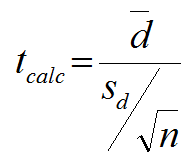# Paired t Test with JMP

### What is the Paired t Test?

A Third type of a Two Sample t-Test is the Paired t Test. This test is used when the two populations are dependent of each other, so each data point from one distribution corresponds to a data point in the other distribution. When using a paired t test, the test statistic is calculated using the average difference between the data pairs, the standard deviation of the differences, and the sample size of either population.

#### Test Statistic

Where:• d is the difference between each pair of data
• is the average of d
• n is the sample size of either population of interest

#### Critical Value

• tcrit is the t value in a Student’s t distribution with the predetermined significance level α and degrees of freedom (n – 1)
• tcrit values for a two-sided and a one-sided t-test with the same significance level α and degrees of freedom (n – 1) are different

The critical t is looked up using the significance level alpha and degrees of freedom (n – 1).

### Use JMP to Run a Paired T-Test

Case study: We are interested to know whether the average salaries (\$1000/yr.) of male and female professors at the same university are the same.

Data File: “PairedT-Test.jmp”

The data were randomly collected from 22 universities. For each university, the salaries of male and female professors were randomly selected.

• Null Hypothesis(H0): μmaleμfemale = 0
• Alternative Hypothesis(Ha): μmaleμfemale ≠ 0

Step 1: Create a new column for the difference between the two data sets of interest.
(MALES – FEMALES = Difference)

Step 2: Test whether the difference is normally distributed.

• Null Hypothesis (H0): The difference between two data sets is normally distributed
• Alternative Hypothesis (Ha): The difference between two data sets is not normally distributed
1. Click Analyze -> Distribution
2. Select “Difference” as “Y, Columns”3. Click “OK”
4. Click on the red triangle button next to “Difference” in the Distribution page
5. Click Continuous Fit -> Normal
6. Click on the red triangle button next to “Fitted Normal”
7. Select “Goodness of Fit”The p-value of the normality test is 0.1336, greater than the alpha level (0.05), so we fail to reject the null hypothesis and we claim that the difference is normally distributed. When the difference is not normally distributed, we need other hypothesis testing methods.

Step 3: Run the paired t-test to compare the means of two dependent data sets.

1. Click Analyze -> Specialized Modeling->Matched Pairs
2. Select both “MALES” and “FEMALES” as “Y, Paired Response”3. Click “OK”Model summary: The p-value of the paired t-test is 0.2127, greater than the alpha level (0.05), so we fail to reject the null hypothesis and we claim that there is no statistically significant difference between the salaries of male and those of female professors. Because the p-value is higher than 0.05, we again fail to reject the null hypothesis, meaning that there is no statistically significant difference between the salaries of male and female professors.